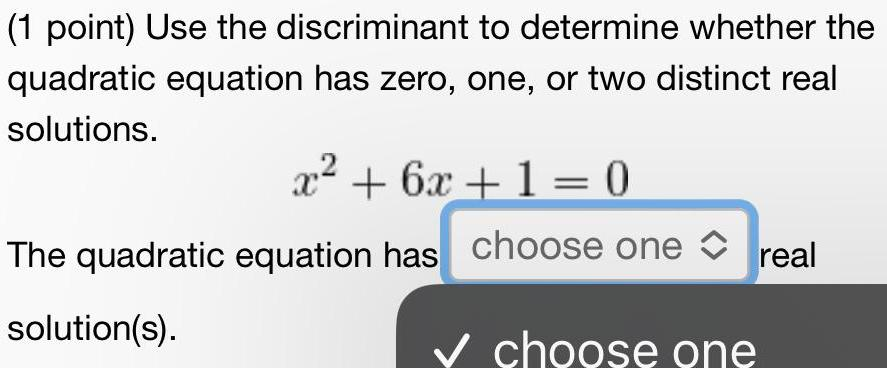Question:

# Use the discriminant to determine whether the quadratic equation has zero, one, or two distinct real solutions.

Last updated: 8/17/2022Use the discriminant to determine whether the quadratic equation has zero, one, or two distinct real solutions. x² + 6x + 1 = 0 The quadratic equation has choose one real solution(s).